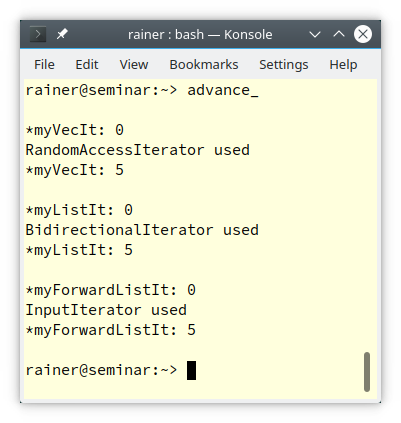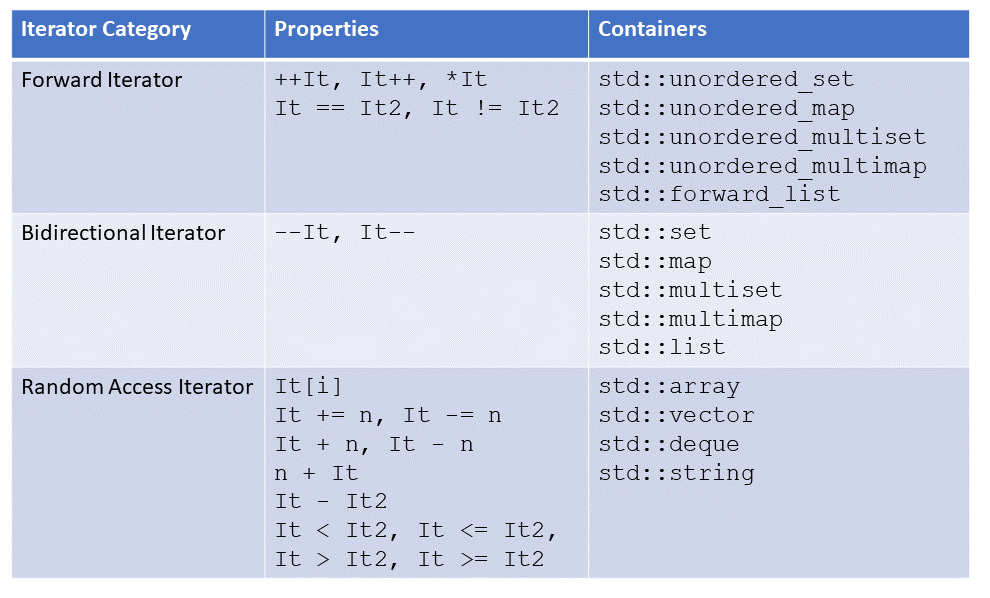# A std::advance Implementation with C++98, C++17, and C++20

In my last post, I presented a possible `std::advance` implementation based on tag dispatching. One of my readers mentioned that I could also implement std::advance based on` constexpr if`, or concepts. His right. So let’s do it.A short reminder:` std::advance(it, n)` increments a given iterator` it` by `n` elements. If` n` is negative, the iterator is decremented. Depending on the container and the iterator provided by the container, a fine-tailored version `std::advance` is used. The reason for this fine-tailored strategy is twofold: type safety and performance. In my last post, “Software Design with Traits and Tag Dispatching“, I implemented my version `std::advance` based on tag dispatching. Before I dive into a possible `std::advance` implementation based on constexpr if (C++17) or concepts (C++20), I want to show once more the tag dispatching (C++98) implementation.

## Tag Dispatching (C++98)

I call the function `advance_` to distinguish it from `std::advance`.

```// advance_.cpp

#include <iterator>
#include <forward_list>
#include <list>
#include <vector>
#include <iostream>

template <typename InputIterator, typename Distance>
void advance_impl(InputIterator& i, Distance n, std::input_iterator_tag) {
std::cout << "InputIterator used" << '\n';
if (n >= 0) { while (n--) ++i; }
}

template <typename BidirectionalIterator, typename Distance>
void advance_impl(BidirectionalIterator& i, Distance n, std::bidirectional_iterator_tag) {
std::cout << "BidirectionalIterator used" << '\n';
if (n >= 0)
while (n--) ++i;
else
while (n++) --i;
}

template <typename RandomAccessIterator, typename Distance>
void advance_impl(RandomAccessIterator& i, Distance n, std::random_access_iterator_tag) {
std::cout << "RandomAccessIterator used" << '\n';
i += n;                                                             // (5)
}

template <typename InputIterator, typename Distance>                    // (4)
void advance_(InputIterator& i, Distance n) {
typename std::iterator_traits<InputIterator>::iterator_category category;
}

int main(){

std::cout << '\n';

std::vector<int> myVec{0, 1, 2, 3, 4, 5, 6, 7, 8, 9};               // (1)
auto myVecIt = myVec.begin();
std::cout << "*myVecIt: " << *myVecIt << '\n';
std::cout << "*myVecIt: " << *myVecIt << '\n';

std::cout << '\n';

std::list<int> myList{0, 1, 2, 3, 4, 5, 6, 7, 8, 9};                // (2)
auto myListIt = myList.begin();
std::cout << "*myListIt: " << *myListIt << '\n';
std::cout << "*myListIt: " << *myListIt << '\n';

std::cout << '\n';

std::forward_list<int> myForwardList{0, 1, 2, 3, 4, 5, 6, 7, 8, 9}; // (3)
auto myForwardListIt = myForwardList.begin();
std::cout << "*myForwardListIt: " << *myForwardListIt << '\n';
std::cout << "*myForwardListIt: " << *myForwardListIt << '\n';

std::cout << '\n';

}
```

Without further ado. Here is the output of the program.

##Modernes C++ Mentoring

Be part of my mentoring programs:

• "Fundamentals for C++ Professionals" (open)
• "Design Patterns and Architectural Patterns with C++" (open)
• "C++20: Get the Details" (reopens December 2023)
• Do you want to stay informed about my mentoring programs: Subscribe via E-Mail.Read my previous post, “Software Design with Traits and Tag Dispatching” to know the details.

## `constexpr if` (C++17)

`constexpr if` enables it to compile source code conditionally.

```template <typename T>
auto getValue(T t) {
if constexpr (std::is_pointer_v<T>)      // (1)
return *t; // deduces return type to int for T = int*
else                                     // (2)
return t;  // deduces return type to int for T = int
}
```

The expression in constexpr if has to be a compile-time predicate. A compile-time predicate is a function that returns a boolean and runs at compile time. I use, in this case, the type-traits function `std::is_pointer.` Both branches have (lines 1 and 2) to be valid.

The following implementation of` std::advance` is a copy from cppreference.com. I only renamed the function to` advance_` to match the function name in my previous program` advance_.cpp,` and added a few line markers. Consequentially, you can replace the previous C++98-based implementation with the following one:

```// implementation via constexpr if, available in C++17
template<class It, class Distance>
constexpr void advance_(It& it, Distance n)
{
using category = typename std::iterator_traits<It>::iterator_category;           // (1)
static_assert(std::is_base_of_v<std::input_iterator_tag, category>);             // (2)

auto dist = typename std::iterator_traits<It>::difference_type(n);               // (3)
if constexpr (std::is_base_of_v<std::random_access_iterator_tag, category>)      // (4)
it += dist;
else {
while (dist > 0) {                                                           // (6)
--dist;
++it;
}
if constexpr (std::is_base_of_v<std::bidirectional_iterator_tag, category>) { // (5)
while (dist < 0) {
++dist;
--it;
}
}
}
}
```

This implementation determines the iterator category based on the used iterator (line 1) and asserts that the iterator category is derived from `std::input_iterator_tag` (line 2). Line 3 determines the value for incrementing the iterator. Now,` constexpr if` it comes into play.  Depending on the type of the iterator, line (4), line (5), or line (6) is used. Line (4) requires `std::random_access_iterator,` line (5) `std::bidirectional_iterator`, and line (6) takes the remaining iterators.

The following graphic shows which container triggers the compilation of which `constexpr if` branch:Honestly, the C++98 version based on tag dispatching is easier to understand. Let me jump one more three years into the future and implement `advance` using concepts.

## Concepts (C++20)

C++20 supports the following concepts for iterators:

```std::input_or_output_iterator
std::input_iterator
std::output_iterator
std::forward_iterator
std::bidirectional_iterator
std::random_access_iterator
std::contiguous_iterator
```

A `std::input_output_iterator` support the operations `++It, It++` , and` *It. std::input_iterator` and `std::output_iterator` are already `std::input_or_output_iterator.` The following relations hold: A contiguous iterator is a random-access iterator, a random-access iterator is a bidirectional iterator, and a bidirectional iterator is a forward iterator. A contiguous iterator requires that the container elements are stored contiguously in memory.

Thanks to concepts, the implementation of advance_ is pretty straightforward. I overload advance_ on the concepts and use concepts as restricted type parameters.

```// conceptsAdvance.cpp

#include <concepts>
#include <iostream>
#include <forward_list>
#include <list>
#include <vector>

template<std::input_iterator I>                              // (1)
std::cout << "InputIterator used" << '\n';
if (n >= 0) { while (n--) ++i; }
}

template<std::bidirectional_iterator I>                       // (2)
std::cout << "BidirectionalIterator used" << '\n';
if (n >= 0)
while (n--) ++i;
else
while (n++) --i;
}

template<std::random_access_iterator I>                       // (3)
std::cout << "RandomAccessIterator used" << '\n';
i += n;
}

int main() {

std::cout << '\n';

std::forward_list forwList{1, 2, 3};
std::forward_list<int>::iterator itFor = forwList.begin();

std::list li{1, 2, 3};
std::list<int>::iterator itBi = li.begin();

std::vector vec{1, 2, 3};
std::vector<int>::iterator itRa = vec.begin();

std::cout << '\n';
}
```

The three variations of the function` advance_` are overloaded with the concepts` std::input_iterator` (line 1),` std::bidirectional_iterator` (line 2), and `std::random_access_iterator` (line 3). The compiler chooses the best-fitting overload. This means that for a` std::forward_list` (line 4) the overload based on the concept` std::forward_iterator`, for a `std::list` (line 5) the overload based on the concept` std::bidirectional_iterator`, and for a` std::vector` (line 6) the overload based on the concept `std::random_access_iterator` is used.

For completeness, here is the program executed with Compiler Explorer.I don’t know which version of advance_ you prefer. The tag dispatching-based C++98 implementation, the constexpr if based C++17 implementation, or the concepts-based C++20 implementation. The concepts-based version is my favorite from a readability and maintainability point of view. The disadvantage is that you need a C++20 compiler. cppreference.com provides you insight into the C++ compiler support of your C++ compiler.

## What’s next?

After this short interplay with the advance algorithm, I bridge in my next post dynamic polymorphism (object orientation) with static polymorphism (templates) to introduce a pretty sophisticated technique: type erasure.

Do you look for new C++ developer job opportunities? Give Jooble a try.

Thanks a lot to my Patreon Supporters: Matt Braun, Roman Postanciuc, Tobias Zindl, G Prvulovic, Reinhold Dröge, Abernitzke, Frank Grimm, Sakib, Broeserl, António Pina, Sergey Agafyin, Андрей Бурмистров, Jake, GS, Lawton Shoemake, Jozo Leko, John Breland, Venkat Nandam, Jose Francisco, Douglas Tinkham, Kuchlong Kuchlong, Robert Blanch, Truels Wissneth, Kris Kafka, Mario Luoni, Friedrich Huber, lennonli, Pramod Tikare Muralidhara, Peter Ware, Daniel Hufschläger, Alessandro Pezzato, Bob Perry, Satish Vangipuram, Andi Ireland, Richard Ohnemus, Michael Dunsky, Leo Goodstadt, John Wiederhirn, Yacob Cohen-Arazi, Florian Tischler, Robin Furness, Michael Young, Holger Detering, Bernd Mühlhaus, Matthieu Bolt, Stephen Kelley, Kyle Dean, Tusar Palauri, Dmitry Farberov, Juan Dent, George Liao, Daniel Ceperley, Jon T Hess, Stephen Totten, Wolfgang Fütterer, Matthias Grün, Phillip Diekmann, Ben Atakora, Ann Shatoff, Rob North, Bhavith C Achar, and Marco Parri Empoli.

Thanks, in particular, to Jon Hess, Lakshman, Christian Wittenhorst, Sherhy Pyton, Dendi Suhubdy, Sudhakar Belagurusamy, Richard Sargeant, Rusty Fleming, John Nebel, Mipko, Alicja Kaminska, Slavko Radman, and David Poole.

 My special thanks to EmbarcaderoMy special thanks to PVS-StudioMy special thanks to Tipi.buildMy special thanks to Take Up Code## Seminars

I’m happy to give online seminars or face-to-face seminars worldwide. Please call me if you have any questions.

### Standard Seminars (English/German)

Here is a compilation of my standard seminars. These seminars are only meant to give you a first orientation.

• C++ – The Core Language
• C++ – The Standard Library
• C++ – Compact
• C++11 and C++14
• Concurrency with Modern C++
• Design Pattern and Architectural Pattern with C++
• Embedded Programming with Modern C++
• Generic Programming (Templates) with C++

#### New

• Clean Code with Modern C++
• C++20

### Contact Me

Modernes C++ Mentoring,Tags:
0 replies